## Section Exercises

1. Describe what it means if there is a model breakdown when using a linear model.

2. What is interpolation when using a linear model?

3. What is extrapolation when using a linear model?

4. Explain the difference between a positive and a negative correlation coefficient.

5. Explain how to interpret the absolute value of a correlation coefficient.

6. A regression was run to determine whether there is a relationship between hours of TV watched per day (x) and number of sit-ups a person can do (y). The results of the regression are given below. Use this to predict the number of sit-ups a person who watches 11 hours of TV can do.

$\begin{cases}y=ax+b\\ a=-1.341\\ b=32.234\\ r=-0.896\end{cases}$

7. A regression was run to determine whether there is a relationship between the diameter of a tree (x, in inches) and the tree’s age (y, in years). The results of the regression are given below. Use this to predict the age of a tree with diameter 10 inches.

$\begin{cases}y=ax+b\\ a=6.301\\ b=-1.044\\ r=-0.970\end{cases}$

For the following exercises, draw a scatter plot for the data provided. Does the data appear to be linearly related?

8.

 0 2 4 6 8 10 –22 –19 –15 –11 –6 –2

9.

 1 2 3 4 5 6 46 50 59 75 100 136

10.

 100 250 300 450 600 750 12 12.6 13.1 14 14.5 15.2

11.

 1 3 5 7 9 11 1 9 28 65 125 216

12. For the following data, draw a scatter plot. If we wanted to know when the population would reach 15,000, would the answer involve interpolation or extrapolation? Eyeball the line, and estimate the answer.

 Year Population 1990 11,500 1995 12,100 2000 12,700 2005 13,000 2010 13,750

13. For the following data, draw a scatter plot. If we wanted to know when the temperature would reach 28 °F, would the answer involve interpolation or extrapolation? Eyeball the line and estimate the answer.

 Temperature, °F 16 18 20 25 30 Time, seconds 46 50 54 55 62

For the following exercises, match each scatterplot with one of the four specified correlations below.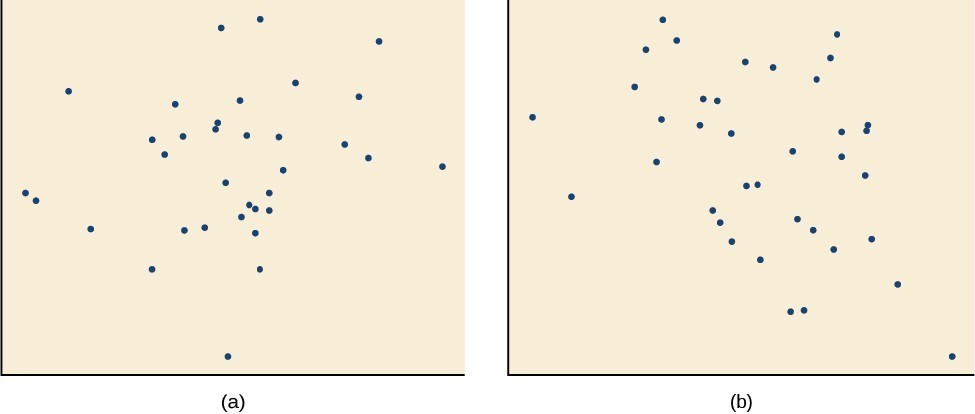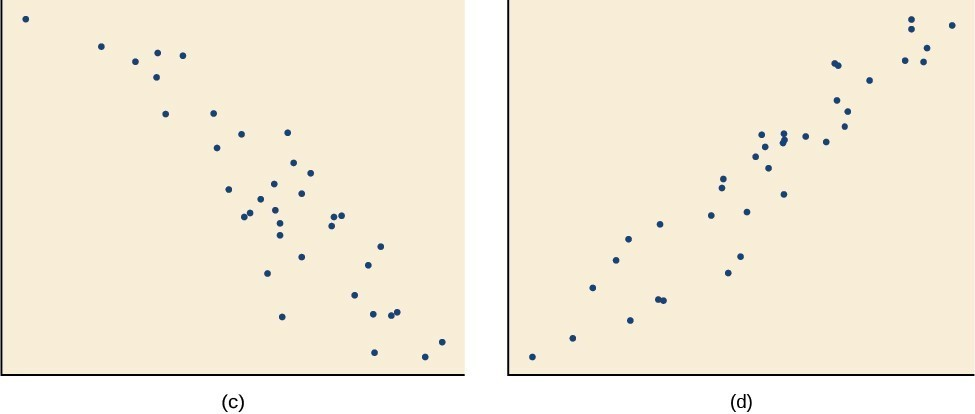14. $r=0.\text{95}$

15. $r=-0.\text{89}$

16. $r=0.26$

17. $r=-0.39$

For the following exercises, draw a best-fit line for the plotted data.

18.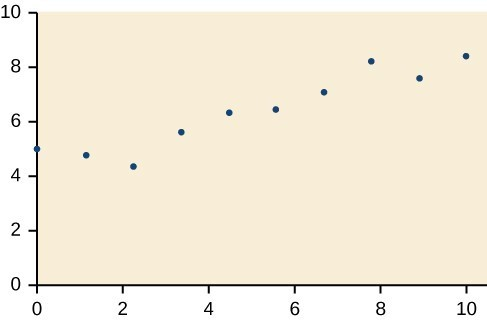19.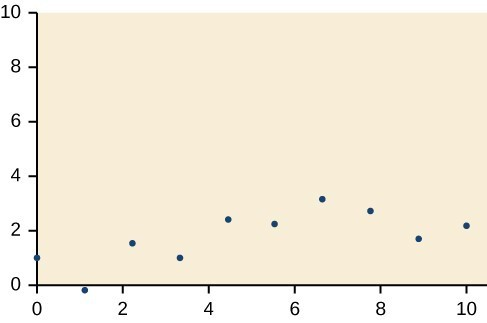20.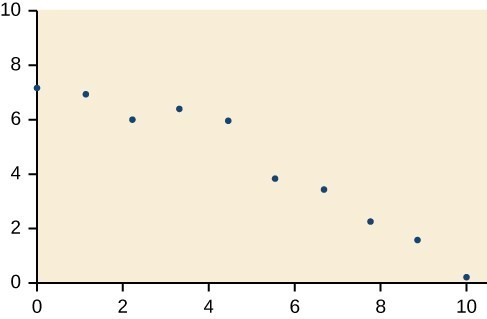21.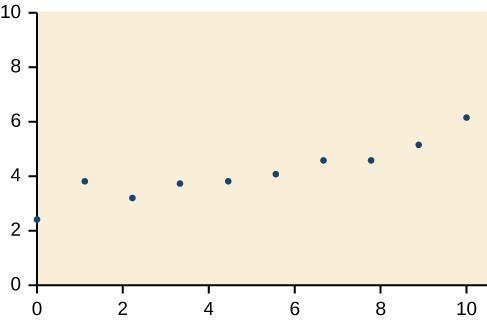22. The U.S. Census tracks the percentage of persons 25 years or older who are college graduates. That data for several years is given in the table below. Determine whether the trend appears linear. If so, and assuming the trend continues, in what year will the percentage exceed 35%?

 Year Percent Graduates 1990 21.3 1992 21.4 1994 22.2 1996 23.6 1998 24.4 2000 25.6 2002 26.7 2004 27.7 2006 28 2008 29.4

23. The U.S. import of wine (in hectoliters) for several years is given below. Determine whether the trend appears linear. If so, and assuming the trend continues, in what year will imports exceed 12,000 hectoliters?

 Year Imports 1992 2665 1994 2688 1996 3565 1998 4129 2000 4584 2002 5655 2004 6549 2006 7950 2008 8487 2009 9462

24. The table below shows the year and the number of people unemployed in a particular city for several years. Determine whether the trend appears linear. If so, and assuming the trend continues, in what year will the number of unemployed reach 5?

 Year Number Unemployed 1990 750 1992 670 1994 650 1996 605 1998 550 2000 510 2002 460 2004 420 2006 380 2008 320

For the following exercises, use each set of data to calculate the regression line using a calculator or other technology tool, and determine the correlation coefficient to 3 decimal places of accuracy.

25.

 x 8 15 26 31 56 y 23 41 53 72 103

26.

 x 5 7 10 12 15 y 4 12 17 22 24

27.

 x y x y 3 21.9 11 15.76 4 22.22 12 13.68 5 22.74 13 14.1 6 22.26 14 14.02 7 20.78 15 11.94 8 17.6 16 12.76 9 16.52 17 11.28 10 18.54 18 9.1

28.

 x y 4 44.8 5 43.1 6 38.8 7 39 8 38 9 32.7 10 30.1 11 29.3 12 27 13 25.8

29.

 x y 21 17 25 11 30 2 31 −1 40 −18 50 −40

30.

 x y 100 2000 80 1798 60 1589 55 1580 40 1390 20 1202

31.

 x y 900 70 988 80 1000 82 1010 84 1200 105 1205 108

32. Graph $f\left(x\right)=0.5x+10$ . Pick a set of 5 ordered pairs using inputs $x=\text{-2},\text{ 1},\text{ 5},\text{ 6},\text{ 9}$ and use linear regression to verify that the function is a good fit for the data.

33. Graph $f\left(x\right)=-2x - 10$. Pick a set of 5 ordered pairs using inputs $x=\text{-2},\text{ 1},\text{ 5},\text{ 6},\text{ 9}$ and use linear regression to verify the function.

For the following exercises, consider this scenario: The profit of a company decreased steadily over a ten-year span. The following ordered pairs shows dollars and the number of units sold in hundreds and the profit in thousands of over the ten-year span, (number of units sold, profit) for specific recorded years:

$\left(\text{46},\text{ 1},\text{6}00\right), \left(\text{48},\text{ 1},\text{55}0\right), \left(\text{5}0,\text{ 1},\text{5}0\text{5}\right), \left(\text{52},\text{ 1},\text{54}0\right), \left(\text{54},\text{ 1},\text{495}\right)$ .

34. Use linear regression to determine a function where the profit in thousands of dollars depends on the number of units sold in hundreds.

35. Find to the nearest tenth and interpret the x-intercept.

36. Find to the nearest tenth and interpret the y-intercept.

For the following exercises, consider this scenario: The population of a city increased steadily over a ten-year span. The following ordered pairs shows the population and the year over the ten-year span, (population, year) for specific recorded years:

(2500, 2000), (2650, 2001), (3000, 2003), (3500, 2006), (4200, 2010)

37. Use linear regression to determine a function y, where the year depends on the population. Round to three decimal places of accuracy.

38. Predict when the population will hit 8,000.

For the following exercises, consider this scenario: The profit of a company increased steadily over a ten-year span. The following ordered pairs show the number of units sold in hundreds and the profit in thousands of over the ten year span, (number of units sold, profit) for specific recorded years:

(46, 250),(48, 305),(50, 350),(52, 390),(54, 410)

39. Use linear regression to determine a function y, where the profit in thousands of dollars depends on the number of units sold in hundreds.

40. Predict when the profit will exceed one million dollars.

For the following exercises, consider this scenario: The profit of a company decreased steadily over a ten-year span. The following ordered pairs show dollars and the number of units sold in hundreds and the profit in thousands of over the ten-year span (number of units sold, profit) for specific recorded years:

(46, 250), (48, 225), (50, 205), (52, 180), (54, 165).

41. Use linear regression to determine a function y, where the profit in thousands of dollars depends on the number of units sold in hundreds.

42. Predict when the profit will dip below the $25,000 threshold. 43. Determine whether the algebraic equation is linear. $2x+3y=7$ 44. Determine whether the algebraic equation is linear. $6{x}^{2}-y=5$ 45. Determine whether the function is increasing or decreasing. $f\left(x\right)=7x - 2$ 46. Determine whether the function is increasing or decreasing. $g\left(x\right)=-x+2$ 47. Given each set of information, find a linear equation that satisfies the given conditions, if possible. Passes through $\left(\text{7},\text{5}\right)$ and $\left(\text{3},\text{17}\right)$ 48. Given each set of information, find a linear equation that satisfies the given conditions, if possible. x-intercept at $\left(\text{6},0\right)$ and y-intercept at $\left(0,\text{1}0\right)$ 49. Find the slope of the line shown in the line graph.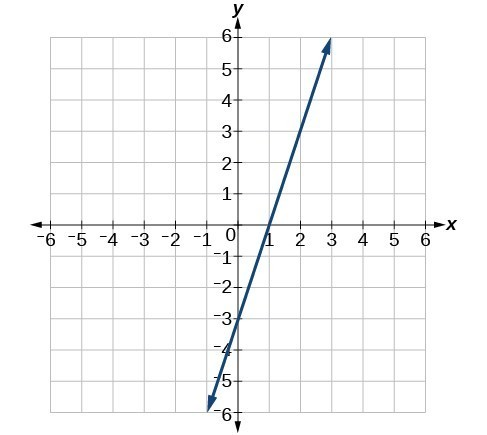50. Find the slope of the line graphed.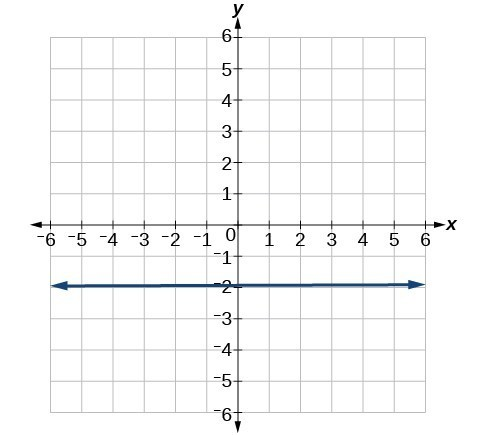51. Write an equation in slope-intercept form for the line shown.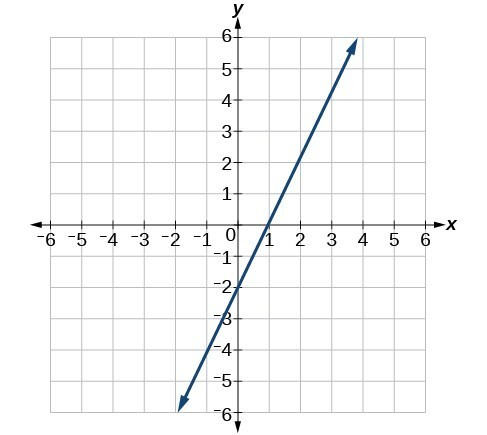52. Does the following table represent a linear function? If so, find the linear equation that models the data.  x –4 0 2 10 g(x) 18 –2 –12 –52 53. Does the following table represent a linear function? If so, find the linear equation that models the data.  x 6 8 12 26 g(x) –8 –12 –18 –46 54. On June 1st, a company has$4,000,000 profit. If the company then loses 150,000 dollars per day thereafter in the month of June, what is the company’s profit nth day after June 1st?

For the following exercises, determine whether the lines given by the equations below are parallel, perpendicular, or neither parallel nor perpendicular:

55. $\begin{cases}2x - 6y=12\hfill \\ -x+3y=1\hfill \end{cases}$

56. $\begin{cases} y=\frac{1}{3}x - 2\hfill \\ 3x+y=-9\hfill \end{cases}$

For the following exercises, find the x– and y– intercepts of the given equation

57. $7x+9y=-63$

58. $f\left(x\right)=2x - 1$

For the following exercises, use the descriptions of the pairs of lines to find the slopes of Line 1 and Line 2. Is each pair of lines parallel, perpendicular, or neither?

59. Line 1: Passes through $\left(5,11\right)$ and $\left(10,1\right)$
Line 2: Passes through $\left(-1,3\right)$ and $\left(-5,11\right)$

60. Line 1: Passes through $\left(8,-10\right)$ and $\left(0,-26\right)$
Line 2: Passes through $\left(2,5\right)$ and $\left(4,4\right)$

61. Write an equation for a line perpendicular to $f\left(x\right)=5x - 1$ and passing through the point (5, 20).

62. Find the equation of a line with a y- intercept of $\left(0,\text{ }2\right)$ and slope $-\frac{1}{2}$.

63. Sketch a graph of the linear function $f\left(t\right)=2t - 5$.

64. Find the point of intersection for the 2 linear functions: $\begin{cases}x=y+6\\ 2x-y=13\end{cases}$

65. A car rental company offers two plans for renting a car.

Plan A: 25 dollars per day and 10 cents per mile
Plan B: 50 dollars per day with free unlimited mileage

How many miles would you need to drive for plan B to save you money?

66. Find the area of a triangle bounded by the y axis, the line $f\left(x\right)=10 - 2x$ , and the line perpendicular to f that passes through the origin.

67. A town’s population increases at a constant rate. In 2010 the population was 55,000. By 2012 the population had increased to 76,000. If this trend continues, predict the population in 2016.

68. The number of people afflicted with the common cold in the winter months dropped steadily by 50 each year since 2004 until 2010. In 2004, 875 people were inflicted. Find the linear function that models the number of people afflicted with the common cold C as a function of the year, t. When will no one be afflicted?

For the following exercises, use the graph in Figure 1 showing the profit, y, in thousands of dollars, of a company in a given year, x, where x represents years since 1980.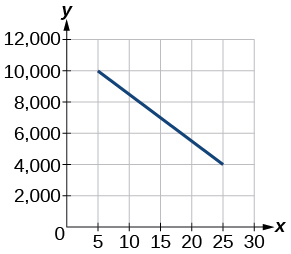Figure 1

69. Find the linear function y, where y depends on x, the number of years since 1980.

70. Find and interpret the y-intercept.

For the following exercise, consider this scenario: In 2004, a school population was 1,700. By 2012 the population had grown to 2,500.

71. Assume the population is changing linearly.
How much did the population grow between the year 2004 and 2012?
What is the average population growth per year?
Find an equation for the population, P, of the school t years after 2004.

For the following exercises, consider this scenario: In 2000, the moose population in a park was measured to be 6,500. By 2010, the population was measured to be 12,500. Assume the population continues to change linearly.

72. Find a formula for the moose population, P.

73. What does your model predict the moose population to be in 2020?

For the following exercises, consider this scenario: The median home values in subdivisions Pima Central and East Valley (adjusted for inflation) are shown in the table below. Assume that the house values are changing linearly.

Year Pima Central East Valley
1970 32,000 120,250
2010 85,000 150,000

74. In which subdivision have home values increased at a higher rate?

75. If these trends were to continue, what would be the median home value in Pima Central in 2015?

76. Draw a scatter plot for the data in the table below. Then determine whether the data appears to be linearly related.

 0 2 4 6 8 10 –105 –50 1 55 105 160

77. Draw a scatter plot for the data in the table below. If we wanted to know when the population would reach 15,000, would the answer involve interpolation or extrapolation?

 Year Population 1990 5,600 1995 5,950 2000 6,300 2005 6,600 2010 6,900

78. Eight students were asked to estimate their score on a 10-point quiz. Their estimated and actual scores are given in the table below. Plot the points, then sketch a line that fits the data.

 Predicted Actual 6 6 7 7 7 8 8 8 7 9 9 10 10 10 10 9

79. Draw a best-fit line for the plotted data.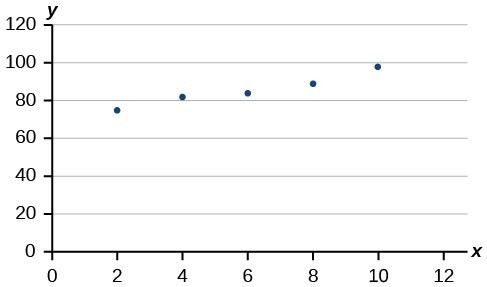For the following exercises, consider the data in the table below, which shows the percent of unemployed in a city of people 25 years or older who are college graduates is given below, by year.

 Year Percent Graduates 2000 6.5 2002 7.0 2005 7.4 2007 8.2 2010 9.0

80. Determine whether the trend appears to be linear. If so, and assuming the trend continues, find a linear regression model to predict the percent of unemployed in a given year to three decimal places.

81. In what year will the percentage exceed 12%?

82. Based on the set of data given below, calculate the regression line using a calculator or other technology tool, and determine the correlation coefficient to three decimal places.

 x 17 20 23 26 29 y 15 25 31 37 40

83. Based on the set of data given below, calculate the regression line using a calculator or other technology tool, and determine the correlation coefficient to three decimal places.

 x 10 12 15 18 20 y 36 34 30 28 22

For the following exercises, consider this scenario: The population of a city increased steadily over a ten-year span. The following ordered pairs show the population and the year over the ten-year span (population, year) for specific recorded years:

(3,600, 2000); (4,000, 2001); (4,700, 2003); (6,000, 2006)

84. Use linear regression to determine a function y, where the year depends on the population, to three decimal places of accuracy.

85. Predict when the population will hit 12,000.

86. What is the correlation coefficient for this model to three decimal places of accuracy?

87. According to the model, what is the population in 2014?